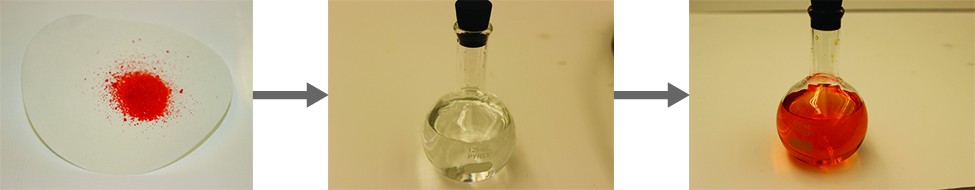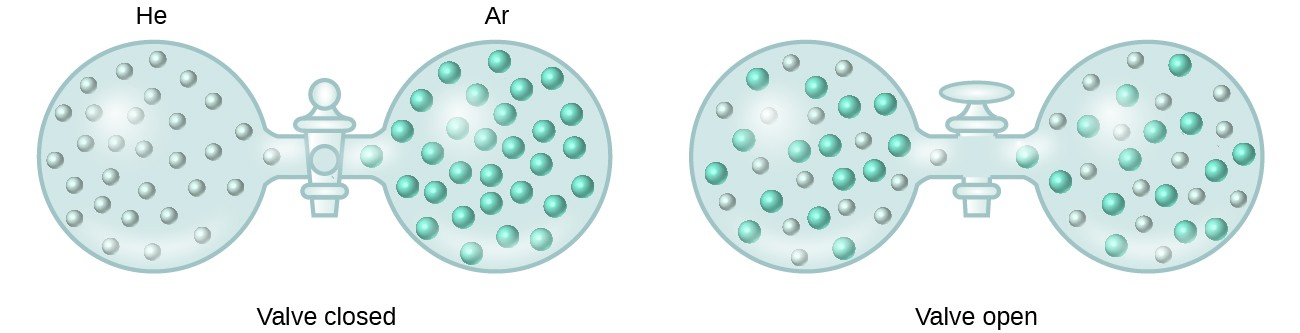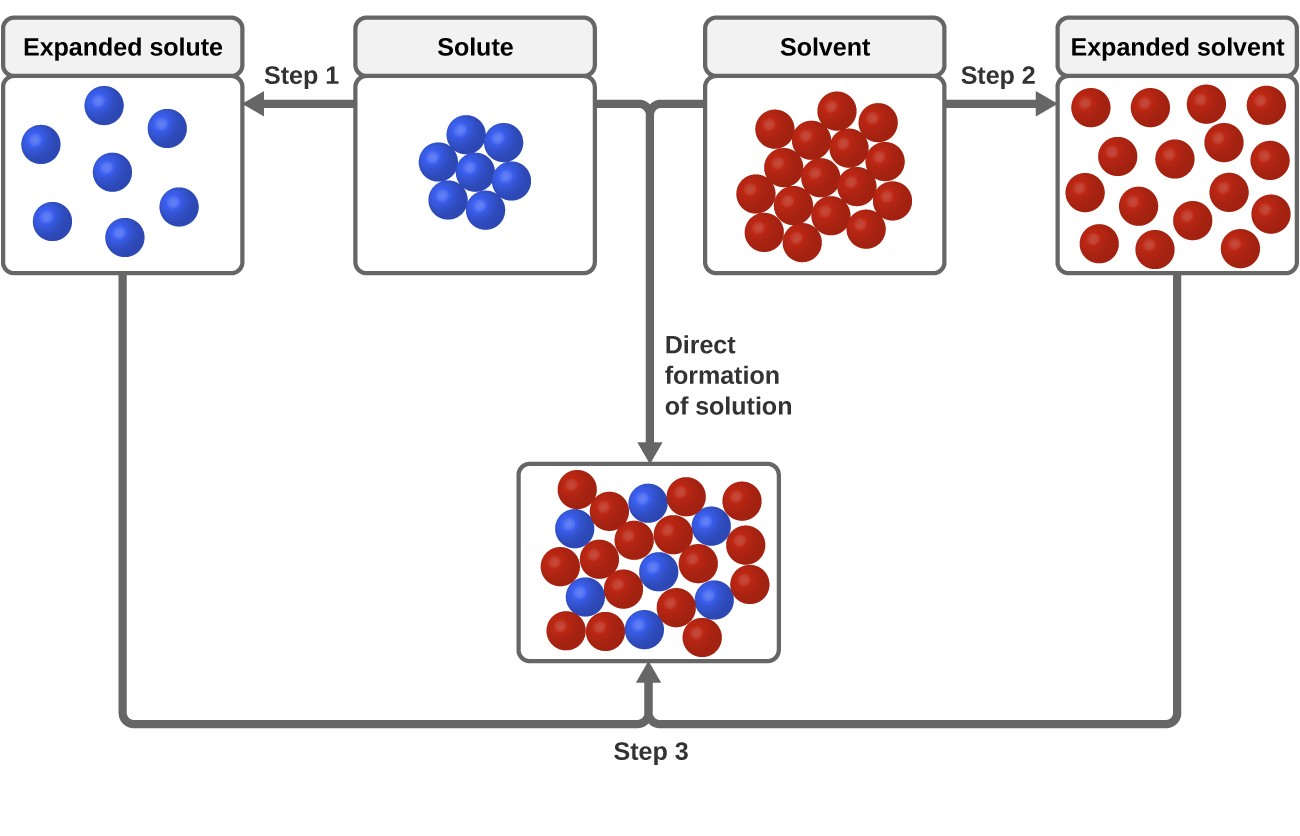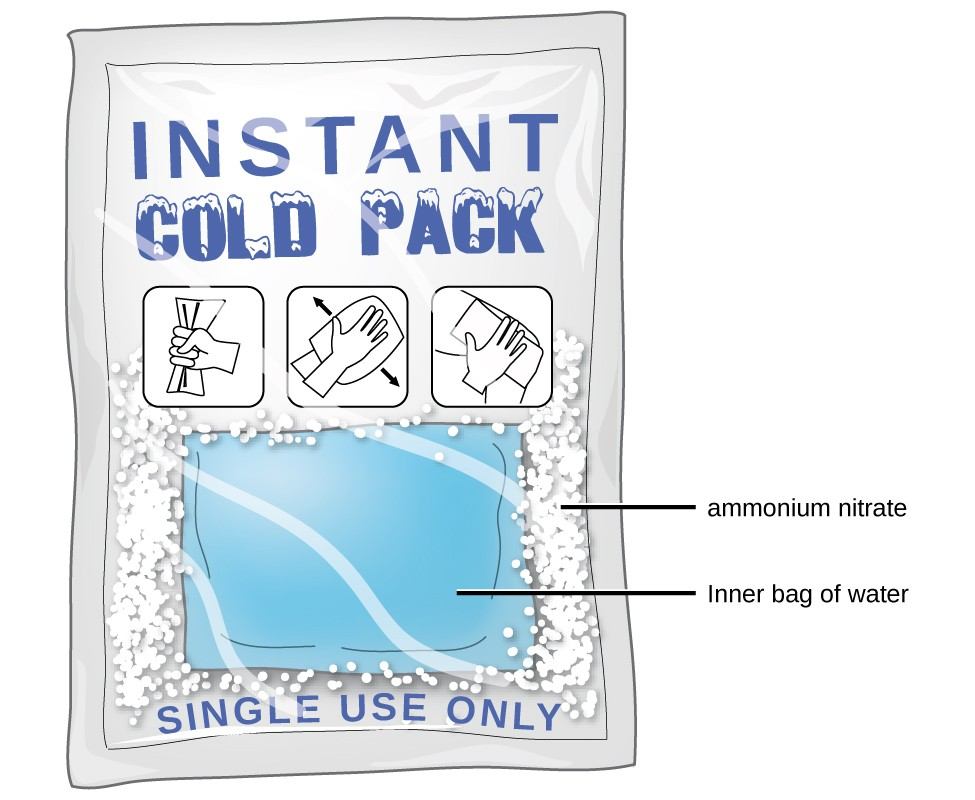## The Dissolution Process

### Learning Outcomes

• Describe the basic properties of solutions and how they form
• Predict whether a given mixture will yield a solution based on molecular properties of its components
• Explain why some solutions either produce or absorb heat when they form

An earlier chapter of this text introduced solutions, defined as homogeneous mixtures of two or more substances. Often, one component of a solution is present at a significantly greater concentration, in which case it is called the solvent. The other components of the solution present in relatively lesser concentrations are called solutes. Sugar is a covalent solid composed of sucrose molecules, C12H22O11. When this compound dissolves in water, its molecules become uniformly distributed among the molecules of water:

${\text{C}}_{12}{\text{H}}_{22}{\text{O}}_{11}\left(s\right)\rightarrow{\text{C}}_{12}{\text{H}}_{22}{\text{O}}_{11}\left(aq\right)$

The subscript “aq” in the equation signifies that the sucrose molecules are solutes and are therefore individually dispersed throughout the aqueous solution (water is the solvent). Although sucrose molecules are heavier than water molecules, they remain dispersed throughout the solution; gravity does not cause them to “settle out” over time.

Potassium dichromate, K2Cr2O7, is an ionic compound composed of colorless potassium ions, K+, and orange dichromate ions, $\text{C}{\text{r}}_{2}{\text{O}}_{7}{}^{2-}\text{.}$ When a small amount of solid potassium chromate is added to water, the compound dissolves and dissociates to yield potassium ions and dichromate ions uniformly distributed throughout the mixture (Figure 1), as indicated in this equation:

${\text{K}}_{2}\text{C}{\text{r}}_{2}{\text{O}}_{7}\left(s\right)\rightarrow 2{\text{K}}^{+}\left(aq\right)+\text{C}{\text{r}}_{2}{\text{O}}_{7}\left(aq\right)$

As for the mixture of sugar and water, this mixture is also an aqueous solution. Its solutes, potassium and dichromate ions, remain individually dispersed among the solvent (water) molecules.Figure 1. When (left) potassium dichromate (K2Cr2O7) is mixed with (middle) water, it forms (right) a homogeneous orange solution. (credit: modifications of work by Mark Ott)

Visit the PhET Sugar and Salt Solutions virtual lab to view simulations of the dissolution of common covalent and ionic substances (sugar and salt) in water.

Water is used so often as a solvent that the word solution has come to imply an aqueous solution to many people. However, almost any gas, liquid, or solid can act as a solvent. Many alloys are solid solutions of one metal dissolved in another; for example, US five-cent coins contain nickel dissolved in copper. Air is a gaseous solution, a homogeneous mixture of nitrogen, oxygen, and several other gases. Oxygen (a gas), alcohol (a liquid), and sugar (a solid) all dissolve in water (a liquid) to form liquid solutions. Table 1 gives examples of several different solutions and the phases of the solutes and solvents.

Table 1. Different Types of Solutions
Solution Solute Solvent
air O2(g) N2(g)
soft drinks CO2(g) H2O(l)
rubbing alcohol H2O(l) C3H8O(l) (2-propanol)
saltwater NaCl(s) H2O(l)
brass Zn(s) Cu(s)

Solutions exhibit these defining traits:

• They are homogeneous; that is, after a solution is mixed, it has the same composition at all points throughout (its composition is uniform).
• The physical state of a solution—solid, liquid, or gas—is typically the same as that of the solvent, as demonstrated by the examples in Table 1.
• The components of a solution are dispersed on a molecular scale; that is, they consist of a mixture of separated molecules, atoms, and/or ions.
• The dissolved solute in a solution will not settle out or separate from the solvent.
• The composition of a solution, or the concentrations of its components, can be varied continuously, within limits.

## The Formation of Solutions

The formation of a solution is an example of a spontaneous process, a process that occurs under specified conditions without the requirement of energy from some external source. Sometimes we stir a mixture to speed up the dissolution process, but this is not necessary; a homogeneous solution would form if we waited long enough. The topic of spontaneity is critically important to the study of chemical thermodynamics and is treated more thoroughly in a later chapter of this text. For purposes of this chapter’s discussion, it will suffice to consider two criteria that favor, but do not guarantee, the spontaneous formation of a solution:

1. a decrease in the internal energy of the system (an exothermic change, as discussed in the previous chapter on thermochemistry)
2. an increase in the disorder in the system (which indicates an increase in the entropy of the system, as you will learn about in the later chapter on thermodynamics)

In the process of dissolution, an internal energy change often, but not always, occurs as heat is absorbed or evolved. An increase in disorder always results when a solution forms.

When the strengths of the intermolecular forces of attraction between solute and solvent species in a solution are no different than those present in the separated components, the solution is formed with no accompanying energy change. Such a solution is called an ideal solution. A mixture of ideal gases (or gases such as helium and argon, which closely approach ideal behavior) is an example of an ideal solution, since the entities comprising these gases experience no significant intermolecular attractions.

When containers of helium and argon are connected, the gases spontaneously mix due to diffusion and form a solution (Figure 2). The formation of this solution clearly involves an increase in disorder, since the helium and argon atoms occupy a volume twice as large as that which each occupied before mixing.Figure 2. Samples of helium and argon spontaneously mix to give a solution in which the disorder of the atoms of the two gases is increased.

Ideal solutions may also form when structurally similar liquids are mixed. For example, mixtures of the alcohols methanol (CH3OH) and ethanol (C2H5OH) form ideal solutions, as do mixtures of the hydrocarbons pentane, C5H12, and hexane, C6H14. Placing methanol and ethanol, or pentane and hexane, in the bulbs shown in Figure 2 will result in the same diffusion and subsequent mixing of these liquids as is observed for the He and Ar gases (although at a much slower rate), yielding solutions with no significant change in energy. Unlike a mixture of gases, however, the components of these liquid-liquid solutions do, indeed, experience intermolecular attractive forces. But since the molecules of the two substances being mixed are structurally very similar, the intermolecular attractive forces between like and unlike molecules are essentially the same, and the dissolution process, therefore, does not entail any appreciable increase or decrease in energy. These examples illustrate how diffusion alone can provide the driving force required to cause the spontaneous formation of a solution. In some cases, however, the relative magnitudes of intermolecular forces of attraction between solute and solvent species may prevent dissolution.

Three types of intermolecular attractive forces are relevant to the dissolution process: solute-solute, solvent-solvent, and solute-solvent. As illustrated in Figure 3, the formation of a solution may be viewed as a stepwise process in which energy is consumed to overcome solute-solute and solvent-solvent attractions (endothermic processes) and released when solute-solvent attractions are established (an exothermic process referred to as solvation). The relative magnitudes of the energy changes associated with these stepwise processes determine whether the dissolution process overall will release or absorb energy. In some cases, solutions do not form because the energy required to separate solute and solvent species is so much greater than the energy released by solvation.Figure 3. This schematic representation of dissolution shows a stepwise process involving the endothermic separation of solute and solvent species (Steps 1 and 2) and exothermic solvation (Step 3).

Consider the example of an ionic compound dissolving in water. Formation of the solution requires the electrostatic forces between the cations and anions of the compound (solute–solute) be overcome completely as attractive forces are established between these ions and water molecules (solute–solvent). Hydrogen bonding between a relatively small fraction of the water molecules must also be overcome to accommodate any dissolved solute. If the solute’s electrostatic forces are significantly greater than the solvation forces, the dissolution process is significantly endothermic and the compound may not dissolve to an appreciable extent. Calcium carbonate, the major component of coral reefs, is one example of such an “insoluble” ionic compound.  On the other hand, if the solvation forces are much stronger than the compound’s electrostatic forces, the dissolution is significantly exothermic and the compound may be highly soluble. A common example of this type of ionic compound is sodium chloride, commonly known as table salt.

As noted at the beginning of this module, spontaneous solution formation is favored, but not guaranteed, by exothermic dissolution processes. While many soluble compounds do, indeed, dissolve with the release of heat, some dissolve endothermically. Ammonium nitrate (NH4NO3) is one such example and is used to make instant cold packs for treating injuries like the one pictured in Figure 4. A thin-walled plastic bag of water is sealed inside a larger bag with solid NH4NO3. When the smaller bag is broken, a solution of NH4NO3 forms, absorbing heat from the surroundings (the injured area to which the pack is applied) and providing a cold compress that decreases swelling. Endothermic dissolutions such as this one require a greater energy input to separate the solute species than is recovered when the solutes are solvated, but they are spontaneous nonetheless due to the increase in disorder that accompanies formation of the solution.Figure 4. An instant cold pack gets cold when certain salts, such as ammonium nitrate, dissolve in water—an endothermic process.

Watch this brief video illustrating endothermic and exothermic dissolution processes. (Note that the video has no narration. You can access the audio description using the widget below the video.)

### Key Concepts and Summary

A solution forms when two or more substances combine physically to yield a mixture that is homogeneous at the molecular level. The solvent is the most concentrated component and determines the physical state of the solution. The solutes are the other components typically present at concentrations less than that of the solvent. Solutions may form endothermically or exothermically, depending upon the relative magnitudes of solute and solvent intermolecular attractive forces. Ideal solutions form with no appreciable change in energy.

### Try It

1. How do solutions differ from compounds? From other mixtures?
2. Which of the principal characteristics of solutions can we see in the solutions of K2Cr2O7 shown in Figure 1?
3. When KNO3 is dissolved in water, the resulting solution is significantly colder than the water was originally.
1. Is the dissolution of KNO3 an endothermic or an exothermic process?
2. What conclusions can you draw about the intermolecular attractions involved in the process?
3. Is the resulting solution an ideal solution?
4. Give an example of each of the following types of solutions:
1. a gas in a liquid
2. a gas in a gas
3. a solid in a solid
5. Indicate the most important types of intermolecular attractions in each of the following solutions:
1. The solution in Figure 1.
2. NO(l) in CO(l)
3. Cl2(g) in Br2(l)
4. HCl(aq) in benzene C6H6(l)
5. Methanol CH3OH(l) in H2O(l)
6. Predict whether each of the following substances would be more soluble in water (polar solvent) or in a hydrocarbon such as heptane (C7H16, nonpolar solvent):
1. vegetable oil (nonpolar)
2. isopropyl alcohol (polar)
3. potassium bromide (ionic)
7. Heat is released when some solutions form; heat is absorbed when other solutions form. Provide a molecular explanation for the difference between these two types of spontaneous processes.
8. Solutions of hydrogen in palladium may be formed by exposing Pd metal to H2 gas. The concentration of hydrogen in the palladium depends on the pressure of H2 gas applied, but in a more complex fashion than can be described by Henry’s law. Under certain conditions, 0.94 g of hydrogen gas is dissolved in 215 g of palladium metal.
1. Determine the molarity of this solution (solution density = 1.8 g/cm3).
2. Determine the molality of this solution (solution density = 1.8 g/cm3).
3. Determine the percent by mass of hydrogen atoms in this solution (solution density = 1.8 g/cm3).

## Glossary

alloy: solid mixture of a metallic element and one or more additional elements

ideal solution: solution that forms with no accompanying energy change

solvation: exothermic process in which intermolecular attractive forces between the solute and solvent in a solution are established

spontaneous process: physical or chemical change that occurs without the addition of energy from an external source

1. If bubbles of gas are observed within the liquid, the mixture is not homogeneous and, thus, not a solution.